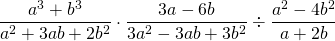Chapter 8: Rational Expressions

# 8.2 Multiplication and Division of Rational Expressions

Multiplying and dividing rational expressions is very similar to the process used to multiply and divide fractions.

Example 8.2.1

Reduce and multiply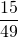and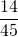.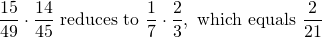(15 and 45 reduce to 1 and 3, and 14 and 49 reduce to 2 and 7)

This process of multiplication is identical to division, except the first step is to reciprocate any fraction that is being divided.

Example 8.2.2

Reduce and divide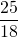by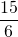.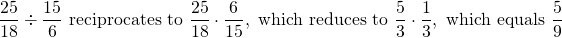(25 and 15 reduce to 5 and 3, and 6 and 18 reduce to 1 and 3)

When multiplying with rational expressions,  follow the same process: first, divide out common factors, then multiply straight across.

Example 8.2.3

Reduce and multiply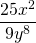and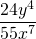.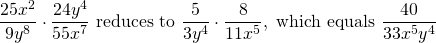(25 and 55 reduce to 5 and 11, 24 and 9 reduce to 8 and 3, x2 and x7 reduce to x5, y4 and y8 reduce to y4)

Remember: when dividing fractions, reciprocate the dividing fraction.

Example 8.2.4

Reduce and divide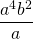by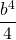.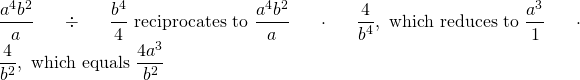(After reciprocating, 4a4b2 and b4 reduce to 4a3 and b2)

In dividing or multiplying some fractions, the polynomials in the fractions must be factored first.

Example 8.2.5

Reduce, factor and multiply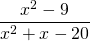and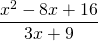.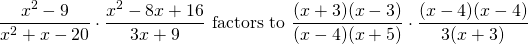Dividing or cancelling out the common factors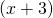and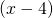leaves us with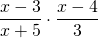, which results in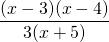.

Example 8.2.6

Reduce, factor and multiply or divide the following fractions: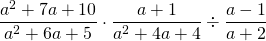Factoring each fraction and reciprocating the last one yields: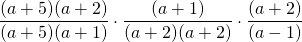Dividing or cancelling out the common polynomials leaves us with: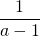# Questions

Simplify each expression.

1.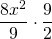2.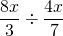3.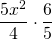4.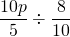5.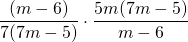6.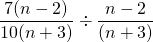7.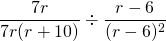8.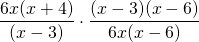9.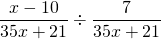10.11.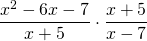12.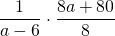13.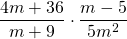14.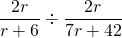15.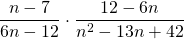16.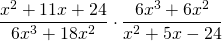17.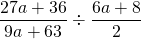18.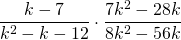19.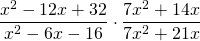20.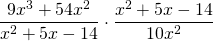21.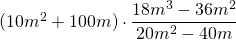22.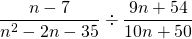23.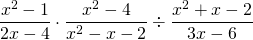24.